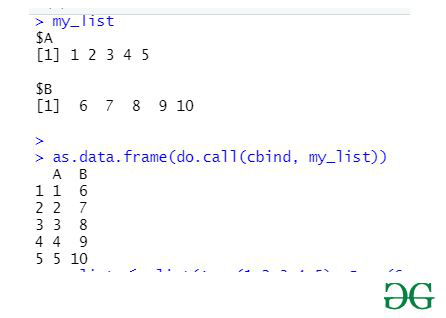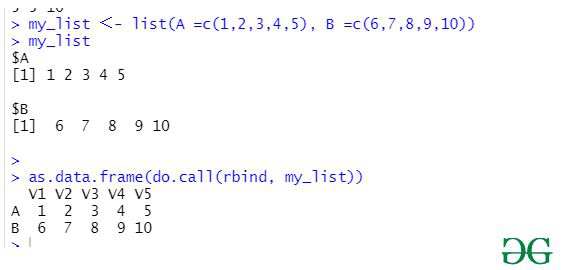# Convert List of Vectors to DataFrame in R

• Last Updated : 09 May, 2021

In this article, we will discuss how to convert the given list of vectors to Dataframe using the help of different functions in the R Programming Language.

For all the methods discussed below, few functions are common because of their functionality. Let us discuss them first.

• as.data.frame() is one of the simplest methods to convert the given list of vectors to the DataFrame in R language with just calling the as.data.frame() function and passing the required parameters, and further this function will be returning the DataFrame converted from the list of vectors given before.

Syntax: as.data.frame(x, row.names = NULL, optional = FALSE, …)

• Further, with the as.data.frame() function user needs to also call the do.call() function which is used here to constructs and executes a function call from a name or a function and a list of arguments to be passed to it.

Syntax: do.call(what, args, quote = FALSE, envir = parent.frame())

Parameters:

• what:-either a function or a non-empty character string naming the function to be called.
• args:a list of arguments to the function call. The names attribute of args gives the argument names.
• quote:-a logical value indicating whether to quote the arguments.
• envir:-an environment within which to evaluate the call.

### Method 1: Using cbind()

cbind() function stands for column-bind. This function can be used to combine several vectors, matrices, or DataFrames by columns.

Syntax: cbind(x1, x2, …, deparse.level = 1)

In this approach, a single vector is considered as one column and then these columns are combined to form a dataframe.

Example:

## R

 `my_list <- ``list``(A =``c``(1,2,3,4,5), ``                ``B =``c``(6,7,8,9,10))`` ` `my_list`` ` `as.data.frame``(``do.call``(cbind, my_list))   `

Output:### Method 2: Using rbind()

rbind() function stands for row-bind. This function can be used to combine several vectors, matrices, or DataFrames by rows.

Syntax: rbind(x1, x2, …, deparse.level = 1)

Parameters:
x1, x2: vector, matrix, DataFrames
deparse.level: This value determines how the column names generated. The default value of deparse.level is 1.

In this approach, each vector is considered a row and then these rows are combined to form a dataframe.

Example:

## R

 `my_list <- ``list``(A =``c``(1,2,3,4,5), ``                ``B =``c``(6,7,8,9,10))`` ` `my_list`` ` `as.data.frame``(``do.call``(rbind, my_list))   `

Output:My Personal Notes arrow_drop_up